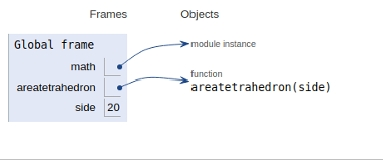# Python Program to calculate the area of a Tetrahedron

In this article, we will learn about the solution and approach to solve the given problem statement.

Problem statement −Given the side of a tetrahedron, we need to find a tetrahedron.

A Tetrahedron is a geometric figure which looks like a pyramid with a triangular base. It is a solid object with four triangular faces, three on the sides, one on the bottom of the base and four vertices or corners.

Here we frame an area function as shown below −

## Example

import math
def areatetrahedron(side):
return (math.sqrt(3) * (side * side))
# Driver Code
side = 20
print("Area of Tetrahedron = ", area_of_tetrahedron(side))

## Output

Area of Tetrahedron = 692.8203230275509

All variables and functions are declared in global scope as shown in the figure below.## Conclusion

In this article, we learned about the approach to find whether it is possible to make a divisible by 3 numbers using all digits in an array.